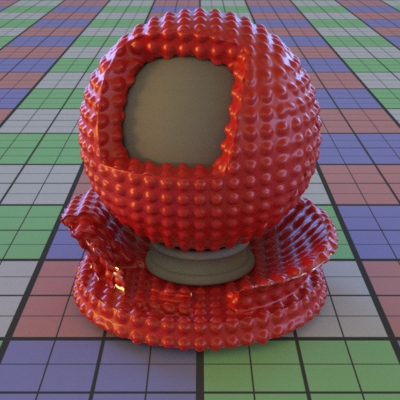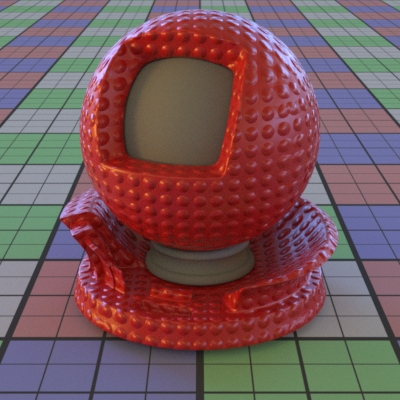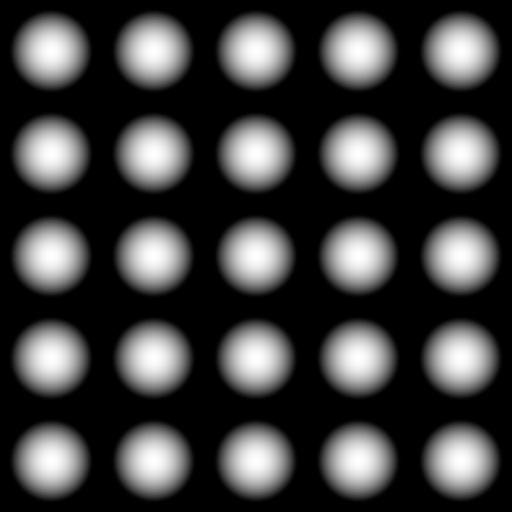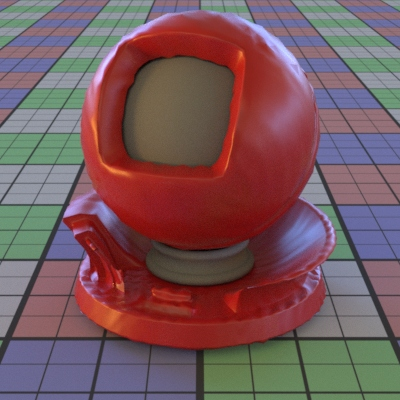# Displacement

## Overview

Displacement maps change the shape of geometry using textures. The geometry is either moved by a scalar amount in the direction of the normal (NormalDisplacement) or an arbitrary direction relative to the normal (VectorDisplacement).

In comparison, normal maps change the direction the geometry surface faces without moving it. If it’s important to change the silhouette of the geometry, you must use a displacment map.Left: normal mapped widget, right: displacement mapped widgetLeft: normal map, right: height map
These textures are applied to the widget example using triplanar mapping

## Assigning Displacement

Displacement is not an input to a material, but part of a layer. This is because mesh displacement happens once, during setup of the render, before the integration (lighting and shading) step.

Here’s what the rdla snippets could look like for creating the corresponding displacement and normal map examples used in this guide:

### Displacement Map RDLA Example

``````-- scene setup, etc
-----------------------------------------------
geoms = {}
assignments = {}

myGeometry = MmGeometry("/myGeometry") {
["model"] = "widget.mm",
["resolution"] = 5, -- subdivision
}
table.insert(geoms, geom)

-- height map creation
myProjection = ProjectTriplanarMap_v2("/myProjection") {
["number of textures"] = "one",
["positive x texture"] = "spheres_height.tx",
["projector"] = myProjectorGeom, -- defined outside example
}

-- turn height map into displacement
myDisplacement = NormalDisplacement("/myDisplacement") {
["height"] = bind(myProjection, 0.1),
["zero_value"] = 0.5,
}

-- displacement is NOT bound in material
myMaterial = DwaSolidDielectricMaterial("/myMaterial") {
["albedo"] = Rgb(0.476, 0.002, 0.002),
["roughness"] = 0.35,
}

-- displacement is bound in layer
table.insert(assignments, {myGeom, "", myMaterial, myLightSet, myDisplacement, undef()})

GeometrySet("/Scene/geometrySet")(geoms)
Layer("/Scene/layer")(assignments)

``````

### Normal Map RDLA Example

``````-- scene setup, etc
-----------------------------------------------
geoms = {}
assignments = {}

myGeometry = MmGeometry("/myGeometry") {
["model"] = "widget.mm",
["resolution"] = 1, -- normal map doesn't need divisions
}
table.insert(geoms, geom)

-- normal map creation
myProjection = ProjectTriplanarNormalMap("/myProjection") {
["number of textures"] = "one",
["positive x texture"] = "spheres_normal.tx",
["projector"] = myProjectorGeom, -- defined outside example
}

-- normal map is bound in material
myMaterial = DwaSolidDielectricMaterial("/myMaterial") {
["albedo"] = Rgb(0.476, 0.002, 0.002),
["roughness"] = 0.35,
["input_normal"] = myProjection,
}

-- layer does not care about normal map
table.insert(assignments, {myGeom, "", myMaterial, myLightSet})

GeometrySet("/Scene/geometrySet")(geoms)
Layer("/Scene/layer")(assignments)

``````

## Mesh Resolution

Displacement is sensitive to mesh resolution. When using displacement, make sure that the mesh is using appropriate tesselation. These options are properties of each mesh in the rdla, and may be adaptive or using basic subdivision.Left: without subdivision, right: with subdivision

## Layering Displacement

Displacement maps of the same type (both NormalDisplacement or both VectorDisplacement) should be layered using OpMap.

CombineDisplacementMap is the utility to create displacement combining both types. It can be used for combining displacements of the same type, but it is less performant than OpMap.